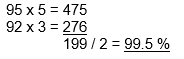Select Page

Each month, a new set of puzzles will be posted.  Come back next month for the solutions and a new set of puzzles, or subscribe to have them sent directly to you.

## MIND-Xpander numeric puzzles

1. If QC = 14, TJ=10 & OK = 4, what does HA = ?
2. What do you get if you divide 40 by a half and add 20 to the result?
3. How can you mathematically combine six 8’s to make 1000?

## Safety-code logic puzzle

To open this safe you must press all the buttons in the correct order. The number on each button tells you how many squares you move and the letters tell you the direction (U = up, D = down, L = left and R = right). Which is the first button you must press to open this safe?## DARTS numeric puzzle

Using 4 darts and the following square dart board, find all combinations to score exactly 150. Numbers/boxes can be used more than once. All darts must be used and each dart must be within one of the numbered boxes. (Note: there are several correct solutions.)## Feedback

There are more than one way of doing these puzzles and may well be more than one answer.  Please let me and others know what alternatives you find by commenting below.  We also welcome general comments on the subject and any feedback you'd like to give.

If you have a question that needs a response from me or you would like to contact me privately, please use the contact form.

## Get more puzzles!

If you've enjoyed doing the puzzles, consider ordering the book; 150+ of the best puzzles in a handy pocket sized format. Click here for full details.

## MIND-Xpander maths puzzles

1. How many hours will a car traveling at 45 mph take to catch up with another car travelling at 30 mph if the slower car starts one hour before the faster car?2. A student needs at least 95% average to receive a grade A. On the first 3 tests, the student averaged 92%. What must the student average on the last 2 tests to receive a grade of A?3. Using the digits 1 to 9, can you form three, three-digit numbers. so that the third number is three times greater than the first and the second number equals the third minus the first?## SQUARE-Sums puzzles

The 4 numbers outside the 9 circles represent the sum of the numbers in the 4 surrounding circles. The numbers in the circles can only be 1 to 9 and each number can be used only once. Three numbers have been provided to get you started. Find the remaining 6 numbers.